# Number theory

Not to be confused with Numerology.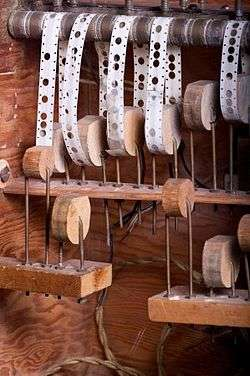A Lehmer sieve, which is a primitive digital computer once used for finding primes and solving simple Diophantine equations.

Number theory or, in older usage, arithmetic is a branch of pure mathematics devoted primarily to the study of the integers. It is sometimes called "The Queen of Mathematics" because of its foundational place in the discipline. Number theorists study prime numbers as well as the properties of objects made out of integers (e.g., rational numbers) or defined as generalizations of the integers (e.g., algebraic integers).

Integers can be considered either in themselves or as solutions to equations (Diophantine geometry). Questions in number theory are often best understood through the study of analytical objects (e.g., the Riemann zeta function) that encode properties of the integers, primes or other number-theoretic objects in some fashion (analytic number theory). One may also study real numbers in relation to rational numbers, e.g., as approximated by the latter (Diophantine approximation).

The older term for number theory is arithmetic. By the early twentieth century, it had been superseded by "number theory".[note 1] (The word "arithmetic" is used by the general public to mean "elementary calculations"; it has also acquired other meanings in mathematical logic, as in Peano arithmetic, and computer science, as in floating point arithmetic.) The use of the term arithmetic for number theory regained some ground in the second half of the 20th century, arguably in part due to French influence.[note 2] In particular, arithmetical is preferred as an adjective to number-theoretic.

## History

### Origins

#### Dawn of arithmetic

The first historical find of an arithmetical nature is a fragment of a table: the broken clay tablet Plimpton 322 (Larsa, Mesopotamia, ca. 1800 BCE) contains a list of "Pythagorean triples", i.e., integerssuch that. The triples are too many and too large to have been obtained by brute force. The heading over the first column reads: "The takiltum of the diagonal which has been subtracted such that the width..."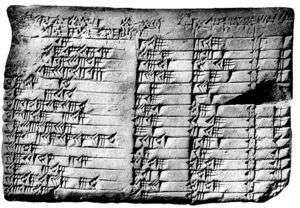The Plimpton 322 tablet

The table's layout suggests that it was constructed by means of what amounts, in modern language, to the identitywhich is implicit in routine Old Babylonian exercises. If some other method was used, the triples were first constructed and then reordered by, presumably for actual use as a "table", i.e., with a view to applications.

It is not known what these applications may have been, or whether there could have been any; Babylonian astronomy, for example, truly flowered only later. It has been suggested instead that the table was a source of numerical examples for school problems.[note 3]

While Babylonian number theory—or what survives of Babylonian mathematics that can be called thus—consists of this single, striking fragment, Babylonian algebra (in the secondary-school sense of "algebra") was exceptionally well developed. Late Neoplatonic sources state that Pythagoras learned mathematics from the Babylonians. Much earlier sources state that Thales and Pythagoras traveled and studied in Egypt.

Euclid IX 21—34 is very probably Pythagorean; it is very simple material ("odd times even is even", "if an odd number measures [= divides] an even number, then it also measures [= divides] half of it"), but it is all that is needed to prove thatis irrational. Pythagorean mystics gave great importance to the odd and the even. The discovery thatis irrational is credited to the early Pythagoreans (pre-Theodorus). By revealing (in modern terms) that numbers could be irrational, this discovery seems to have provoked the first foundational crisis in mathematical history; its proof or its divulgation are sometimes credited to Hippasus, who was expelled or split from the Pythagorean sect. This forced a distinction between numbers (integers and the rationals—the subjects of arithmetic), on the one hand, and lengths and proportions (which we would identify with real numbers, whether rational or not), on the other hand.

The Pythagorean tradition spoke also of so-called polygonal or figurate numbers. While square numbers, cubic numbers, etc., are seen now as more natural than triangular numbers, pentagonal numbers, etc., the study of the sums of triangular and pentagonal numbers would prove fruitful in the early modern period (17th to early 19th century).

We know of no clearly arithmetical material in ancient Egyptian or Vedic sources, though there is some algebra in both. The Chinese remainder theorem appears as an exercise  in Sun Zi's Suan Ching, also known as The Mathematical Classic of Sun Zi (3rd, 4th or 5th century CE.) (There is one important step glossed over in Sun Zi's solution:[note 4] it is the problem that was later solved by Āryabhaṭa's Kuṭṭaka – see below.)

There is also some numerical mysticism in Chinese mathematics,[note 5] but, unlike that of the Pythagoreans, it seems to have led nowhere. Like the Pythagoreans' perfect numbers, magic squares have passed from superstition into recreation.

#### Classical Greece and the early Hellenistic period

Further information: Ancient Greek mathematics

Aside from a few fragments, the mathematics of Classical Greece is known to us either through the reports of contemporary non-mathematicians or through mathematical works from the early Hellenistic period. In the case of number theory, this means, by and large, Plato and Euclid, respectively.

Plato had a keen interest in mathematics, and distinguished clearly between arithmetic and calculation. (By arithmetic he meant, in part, theorising on number, rather than what arithmetic or number theory have come to mean.) It is through one of Plato's dialogues—namely, Theaetetus—that we know that Theodorus had proven thatare irrational. Theaetetus was, like Plato, a disciple of Theodorus's; he worked on distinguishing different kinds of incommensurables, and was thus arguably a pioneer in the study of number systems. (Book X of Euclid's Elements is described by Pappus as being largely based on Theaetetus's work.)

Euclid devoted part of his Elements to prime numbers and divisibility, topics that belong unambiguously to number theory and are basic to it (Books VII to IX of Euclid's Elements). In particular, he gave an algorithm for computing the greatest common divisor of two numbers (the Euclidean algorithm; Elements, Prop. VII.2) and the first known proof of the infinitude of primes (Elements, Prop. IX.20).

In 1773, Lessing published an epigram he had found in a manuscript during his work as a librarian; it claimed to be a letter sent by Archimedes to Eratosthenes. The epigram proposed what has become known as Archimedes' cattle problem; its solution (absent from the manuscript) requires solving an indeterminate quadratic equation (which reduces to what would later be misnamed Pell's equation). As far as we know, such equations were first successfully treated by the Indian school. It is not known whether Archimedes himself had a method of solution.

#### Diophantus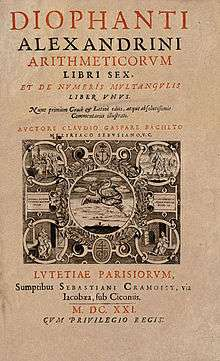Title page of the 1621 edition of Diophantus' Arithmetica, translated into Latin by Claude Gaspard Bachet de Méziriac.

Very little is known about Diophantus of Alexandria; he probably lived in the third century CE, that is, about five hundred years after Euclid. Six out of the thirteen books of Diophantus's Arithmetica survive in the original Greek; four more books survive in an Arabic translation. The Arithmetica is a collection of worked-out problems where the task is invariably to find rational solutions to a system of polynomial equations, usually of the formor. Thus, nowadays, we speak of Diophantine equations when we speak of polynomial equations to which rational or integer solutions must be found.

One may say that Diophantus was studying rational points — i.e., points whose coordinates are rational — on curves and algebraic varieties; however, unlike the Greeks of the Classical period, who did what we would now call basic algebra in geometrical terms, Diophantus did what we would now call basic algebraic geometry in purely algebraic terms. In modern language, what Diophantus did was to find rational parametrizations of varieties; that is, given an equation of the form (say), his aim was to find (in essence) three rational functionssuch that, for all values ofand, settingforgives a solution toDiophantus also studied the equations of some non-rational curves, for which no rational parametrisation is possible. He managed to find some rational points on these curves (elliptic curves, as it happens, in what seems to be their first known occurrence) by means of what amounts to a tangent construction: translated into coordinate geometry (which did not exist in Diophantus's time), his method would be visualised as drawing a tangent to a curve at a known rational point, and then finding the other point of intersection of the tangent with the curve; that other point is a new rational point. (Diophantus also resorted to what could be called a special case of a secant construction.)

While Diophantus was concerned largely with rational solutions, he assumed some results on integer numbers, in particular that every integer is the sum of four squares (though he never stated as much explicitly).

#### Āryabhaṭa, Brahmagupta, Bhāskara

While Greek astronomy probably influenced Indian learning, to the point of introducing trigonometry, it seems to be the case that Indian mathematics is otherwise an indigenous tradition; in particular, there is no evidence that Euclid's Elements reached India before the 18th century.

Āryabhaṭa (476–550 CE) showed that pairs of simultaneous congruences,could be solved by a method he called kuṭṭaka, or pulveriser; this is a procedure close to (a generalisation of) the Euclidean algorithm, which was probably discovered independently in India. Āryabhaṭa seems to have had in mind applications to astronomical calculations.

Brahmagupta (628 CE) started the systematic study of indefinite quadratic equations—in particular, the misnamed Pell equation, in which Archimedes may have first been interested, and which did not start to be solved in the West until the time of Fermat and Euler. Later Sanskrit authors would follow, using Brahmagupta's technical terminology. A general procedure (the chakravala, or "cyclic method") for solving Pell's equation was finally found by Jayadeva (cited in the eleventh century; his work is otherwise lost); the earliest surviving exposition appears in Bhāskara II's Bīja-gaṇita (twelfth century).

Indian mathematics remained largely unknown in Europe until the late eighteenth century; Brahmagupta and Bhāskara's work was translated into English in 1817 by Henry Colebrooke.

#### Arithmetic in the Islamic golden age

Further information: Mathematics in medieval Islam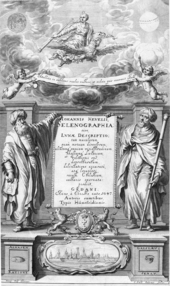Al-Haytham seen by the West: frontispice of Selenographia, showing Alhasen [sic] representing knowledge through reason, and Galileo representing knowledge through the senses.

In the early ninth century, the caliph Al-Ma'mun ordered translations of many Greek mathematical works and at least one Sanskrit work (the Sindhind, which may  or may not be Brahmagupta's Brāhmasphuţasiddhānta). Diophantus's main work, the Arithmetica, was translated into Arabic by Qusta ibn Luqa (820–912). Part of the treatise al-Fakhri (by al-Karajī, 953 – ca. 1029) builds on it to some extent. According to Rashed Roshdi, Al-Karajī's contemporary Ibn al-Haytham knew what would later be called Wilson's theorem.

#### Western Europe in the Middle Ages

Other than a treatise on squares in arithmetic progression by Fibonacci — who lived and studied in north Africa and Constantinople during his formative years, ca. 1175–1200 — no number theory to speak of was done in western Europe during the Middle Ages. Matters started to change in Europe in the late Renaissance, thanks to a renewed study of the works of Greek antiquity. A catalyst was the textual emendation and translation into Latin of Diophantus's Arithmetica (Bachet, 1621, following a first attempt by Xylander, 1575).

### Early modern number theory

#### Fermat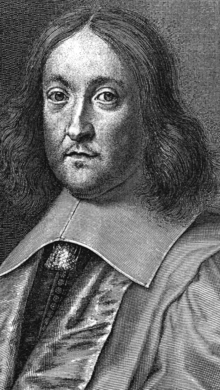Pierre de Fermat

Pierre de Fermat (1601–1665) never published his writings; in particular, his work on number theory is contained almost entirely in letters to mathematicians and in private marginal notes. He wrote down nearly no proofs in number theory; he had no models in the area. He did make repeated use of mathematical induction, introducing the method of infinite descent.

One of Fermat's first interests was perfect numbers (which appear in Euclid, Elements IX) and amicable numbers;[note 6] this led him to work on integer divisors, which were from the beginning among the subjects of the correspondence (1636 onwards) that put him in touch with the mathematical community of the day. He had already studied Bachet's edition of Diophantus carefully; by 1643, his interests had shifted largely to Diophantine problems and sums of squares (also treated by Diophantus).

Fermat's achievements in arithmetic include:

• Fermat's little theorem (1640), stating that, if a is not divisible by a prime p, then[note 7]
• If a and b are coprime, thenis not divisible by any prime congruent to 1 modulo 4; and every prime congruent to 1 modulo 4 can be written in the form. These two statements also date from 1640; in 1659, Fermat stated to Huygens that he had proven the latter statement by the method of infinite descent. Fermat and Frenicle also did some work (some of it erroneous) on other quadratic forms.
• Fermat posed the problem of solvingas a challenge to English mathematicians (1657). The problem was solved in a few months by Wallis and Brouncker. Fermat considered their solution valid, but pointed out they had provided an algorithm without a proof (as had Jayadeva and Bhaskara, though Fermat would never know this.) He states that a proof can be found by descent.
• Fermat developed methods for (doing what in our terms amounts to) finding points on curves of genus 0 and 1. As in Diophantus, there are many special procedures and what amounts to a tangent construction, but no use of a secant construction.
• Fermat states and proves (by descent) in the appendix to Observations on Diophantus (Obs. XLV) thathas no non-trivial solutions in the integers. Fermat also mentioned to his correspondents thathas no non-trivial solutions, and that this could be proven by descent. The first known proof is due to Euler (1753; indeed by descent).

Fermat's claim ("Fermat's last theorem") to have shown there are no solutions tofor all(the only known proof of which is beyond his methods) appears only in his annotations on the margin of his copy of Diophantus; he never claimed this to others and thus would have had no need to retract it if he found any mistake in his supposed proof.

#### Euler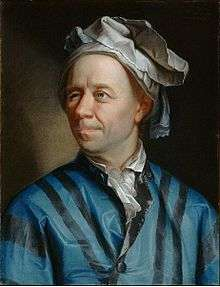Leonhard Euler

The interest of Leonhard Euler (1707–1783) in number theory was first spurred in 1729, when a friend of his, the amateur[note 8] Goldbach, pointed him towards some of Fermat's work on the subject. This has been called the "rebirth" of modern number theory, after Fermat's relative lack of success in getting his contemporaries' attention for the subject. Euler's work on number theory includes the following:

• Proofs for Fermat's statements. This includes Fermat's little theorem (generalised by Euler to non-prime moduli); the fact thatif and only if; initial work towards a proof that every integer is the sum of four squares (the first complete proof is by Joseph-Louis Lagrange (1770), soon improved by Euler himself); the lack of non-zero integer solutions to(implying the case n=4 of Fermat's last theorem, the case n=3 of which Euler also proved by a related method).
• Pell's equation, first misnamed by Euler. He wrote on the link between continued fractions and Pell's equation.
• First steps towards analytic number theory. In his work of sums of four squares, partitions, pentagonal numbers, and the distribution of prime numbers, Euler pioneered the use of what can be seen as analysis (in particular, infinite series) in number theory. Since he lived before the development of complex analysis, most of his work is restricted to the formal manipulation of power series. He did, however, do some very notable (though not fully rigorous) early work on what would later be called the Riemann zeta function.
• Quadratic forms. Following Fermat's lead, Euler did further research on the question of which primes can be expressed in the form, some of it prefiguring quadratic reciprocity. 
• Diophantine equations. Euler worked on some Diophantine equations of genus 0 and 1. In particular, he studied Diophantus's work; he tried to systematise it, but the time was not yet ripe for such an endeavour – algebraic geometry was still in its infancy. He did notice there was a connection between Diophantine problems and elliptic integrals, whose study he had himself initiated.

#### Lagrange, Legendre and Gauss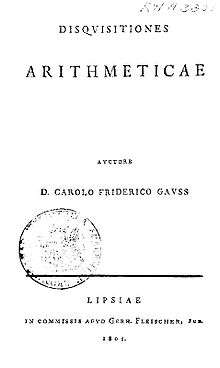Joseph-Louis Lagrange (1736–1813) was the first to give full proofs of some of Fermat's and Euler's work and observations – for instance, the four-square theorem and the basic theory of the misnamed "Pell's equation" (for which an algorithmic solution was found by Fermat and his contemporaries, and also by Jayadeva and Bhaskara II before them.) He also studied quadratic forms in full generality (as opposed to) defining their equivalence relation, showing how to put them in reduced form, etc.

Adrien-Marie Legendre (1752–1833) was the first to state the law of quadratic reciprocity. He also conjectured what amounts to the prime number theorem and Dirichlet's theorem on arithmetic progressions. He gave a full treatment of the equationand worked on quadratic forms along the lines later developed fully by Gauss. In his old age, he was the first to prove "Fermat's last theorem" for(completing work by Peter Gustav Lejeune Dirichlet, and crediting both him and Sophie Germain).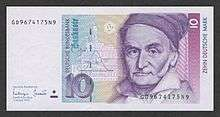Carl Friedrich Gauss

In his Disquisitiones Arithmeticae (1798), Carl Friedrich Gauss (1777–1855) proved the law of quadratic reciprocity and developed the theory of quadratic forms (in particular, defining their composition). He also introduced some basic notation (congruences) and devoted a section to computational matters, including primality tests. The last section of the Disquisitiones established a link between roots of unity and number theory:

The theory of the division of the circle...which is treated in sec. 7 does not belong by itself to arithmetic, but its principles can only be drawn from higher arithmetic.

In this way, Gauss arguably made a first foray towards both Évariste Galois's work and algebraic number theory.

### Maturity and division into subfields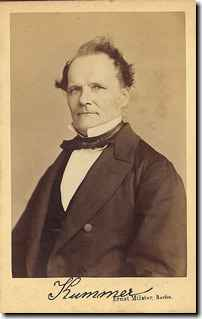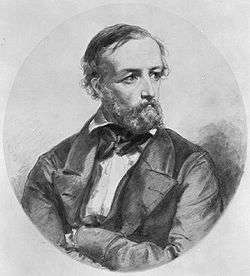Starting early in the nineteenth century, the following developments gradually took place:

• The rise to self-consciousness of number theory (or higher arithmetic) as a field of study.
• The development of much of modern mathematics necessary for basic modern number theory: complex analysis, group theory, Galois theory—accompanied by greater rigor in analysis and abstraction in algebra.
• The rough subdivision of number theory into its modern subfields—in particular, analytic and algebraic number theory.

Algebraic number theory may be said to start with the study of reciprocity and cyclotomy, but truly came into its own with the development of abstract algebra and early ideal theory and valuation theory; see below. A conventional starting point for analytic number theory is Dirichlet's theorem on arithmetic progressions (1837),  whose proof introduced L-functions and involved some asymptotic analysis and a limiting process on a real variable. The first use of analytic ideas in number theory actually goes back to Euler (1730s),  who used formal power series and non-rigorous (or implicit) limiting arguments. The use of complex analysis in number theory comes later: the work of Bernhard Riemann (1859) on the zeta function is the canonical starting point; Jacobi's four-square theorem (1839), which predates it, belongs to an initially different strand that has by now taken a leading role in analytic number theory (modular forms).

The history of each subfield is briefly addressed in its own section below; see the main article of each subfield for fuller treatments. Many of the most interesting questions in each area remain open and are being actively worked on.

## Main subdivisions

### Elementary tools

The term elementary generally denotes a method that does not use complex analysis. For example, the prime number theorem was first proven using complex analysis in 1896, but an elementary proof was found only in 1949 by Erdős and Selberg. The term is somewhat ambiguous: for example, proofs based on complex Tauberian theorems (e.g. Wiener–Ikehara) are often seen as quite enlightening but not elementary, in spite of using Fourier analysis, rather than complex analysis as such. Here as elsewhere, an elementary proof may be longer and more difficult for most readers than a non-elementary one.

Number theory has the reputation of being a field many of whose results can be stated to the layperson. At the same time, the proofs of these results are not particularly accessible, in part because the range of tools they use is, if anything, unusually broad within mathematics.

### Analytic number theory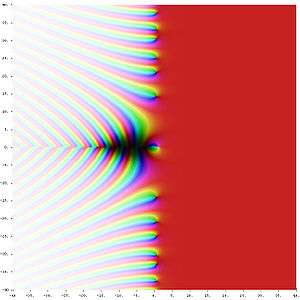Riemann zeta function ζ(s) in the complex plane. The color of a point s gives the value of ζ(s): dark colors denote values close to zero and hue gives the value's argument.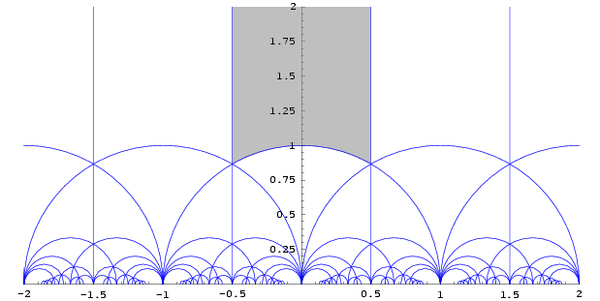The action of the modular group on the upper half plane. The region in grey is the standard fundamental domain.

Analytic number theory may be defined

• in terms of its tools, as the study of the integers by means of tools from real and complex analysis; or
• in terms of its concerns, as the study within number theory of estimates on size and density, as opposed to identities.

Some subjects generally considered to be part of analytic number theory, e.g., sieve theory,[note 9] are better covered by the second rather than the first definition: some of sieve theory, for instance, uses little analysis,[note 10] yet it does belong to analytic number theory.

The following are examples of problems in analytic number theory: the prime number theorem, the Goldbach conjecture (or the twin prime conjecture, or the Hardy–Littlewood conjectures), the Waring problem and the Riemann hypothesis. Some of the most important tools of analytic number theory are the circle method, sieve methods and L-functions (or, rather, the study of their properties). The theory of modular forms (and, more generally, automorphic forms) also occupies an increasingly central place in the toolbox of analytic number theory.

One may ask analytic questions about algebraic numbers, and use analytic means to answer such questions; it is thus that algebraic and analytic number theory intersect. For example, one may define prime ideals (generalizations of prime numbers in the field of algebraic numbers) and ask how many prime ideals there are up to a certain size. This question can be answered by means of an examination of Dedekind zeta functions, which are generalizations of the Riemann zeta function, a key analytic object at the roots of the subject. This is an example of a general procedure in analytic number theory: deriving information about the distribution of a sequence (here, prime ideals or prime numbers) from the analytic behavior of an appropriately constructed complex-valued function.

### Algebraic number theory

An algebraic number is any complex number that is a solution to some polynomial equationwith rational coefficients; for example, every solutionof(say) is an algebraic number. Fields of algebraic numbers are also called algebraic number fields, or shortly number fields. Algebraic number theory studies algebraic number fields. Thus, analytic and algebraic number theory can and do overlap: the former is defined by its methods, the latter by its objects of study.

It could be argued that the simplest kind of number fields (viz., quadratic fields) were already studied by Gauss, as the discussion of quadratic forms in Disquisitiones arithmeticae can be restated in terms of ideals and norms in quadratic fields. (A quadratic field consists of all numbers of the form, whereandare rational numbers andis a fixed rational number whose square root is not rational.) For that matter, the 11th-century chakravala method amounts—in modern terms—to an algorithm for finding the units of a real quadratic number field. However, neither Bhāskara nor Gauss knew of number fields as such.

The grounds of the subject as we know it were set in the late nineteenth century, when ideal numbers, the theory of ideals and valuation theory were developed; these are three complementary ways of dealing with the lack of unique factorisation in algebraic number fields. (For example, in the field generated by the rationals and, the numbercan be factorised both asand; all of,,andare irreducible, and thus, in a naïve sense, analogous to primes among the integers.) The initial impetus for the development of ideal numbers (by Kummer) seems to have come from the study of higher reciprocity laws,i.e., generalisations of quadratic reciprocity.

Number fields are often studied as extensions of smaller number fields: a field L is said to be an extension of a field K if L contains K. (For example, the complex numbers C are an extension of the reals R, and the reals R are an extension of the rationals Q.) Classifying the possible extensions of a given number field is a difficult and partially open problem. Abelian extensions—that is, extensions L of K such that the Galois group[note 11] Gal(L/K) of L over K is an abelian group—are relatively well understood. Their classification was the object of the programme of class field theory, which was initiated in the late 19th century (partly by Kronecker and Eisenstein) and carried out largely in 1900—1950.

An example of an active area of research in algebraic number theory is Iwasawa theory. The Langlands program, one of the main current large-scale research plans in mathematics, is sometimes described as an attempt to generalise class field theory to non-abelian extensions of number fields.

### Diophantine geometry

Main article: Diophantine geometry

The central problem of Diophantine geometry is to determine when a Diophantine equation has solutions, and if it does, how many. The approach taken is to think of the solutions of an equation as a geometric object.

For example, an equation in two variables defines a curve in the plane. More generally, an equation, or system of equations, in two or more variables defines a curve, a surface or some other such object in n-dimensional space. In Diophantine geometry, one asks whether there are any rational points (points all of whose coordinates are rationals) or integral points (points all of whose coordinates are integers) on the curve or surface. If there are any such points, the next step is to ask how many there are and how they are distributed. A basic question in this direction is: are there finitely or infinitely many rational points on a given curve (or surface)? What about integer points?

An example here may be helpful. Consider the Pythagorean equation; we would like to study its rational solutions, i.e., its solutionssuch that x and y are both rational. This is the same as asking for all integer solutions to; any solution to the latter equation gives us a solution,to the former. It is also the same as asking for all points with rational coordinates on the curve described by. (This curve happens to be a circle of radius 1 around the origin.)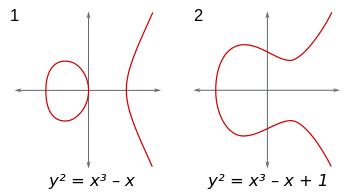Two examples of an elliptic curve, i.e., a curve of genus 1 having at least one rational point. (Either graph can be seen as a slice of a torus in four-dimensional space.)

The rephrasing of questions on equations in terms of points on curves turns out to be felicitous. The finiteness or not of the number of rational or integer points on an algebraic curve—that is, rational or integer solutions to an equation, whereis a polynomial in two variables—turns out to depend crucially on the genus of the curve. The genus can be defined as follows:[note 12] allow the variables into be complex numbers; thendefines a 2-dimensional surface in (projective) 4-dimensional space (since two complex variables can be decomposed into four real variables, i.e., four dimensions). Count the number of (doughnut) holes in the surface; call this number the genus of. Other geometrical notions turn out to be just as crucial.

There is also the closely linked area of Diophantine approximations: given a number, how well can it be approximated by rationals? (We are looking for approximations that are good relative to the amount of space that it takes to write the rational: call(with) a good approximation toif, whereis large.) This question is of special interest ifis an algebraic number. Ifcannot be well approximated, then some equations do not have integer or rational solutions. Moreover, several concepts (especially that of height) turn out to be crucial both in Diophantine geometry and in the study of Diophantine approximations. This question is also of special interest in transcendental number theory: if a number can be better approximated than any algebraic number, then it is a transcendental number. It is by this argument that π and e have been shown to be transcendental.

Diophantine geometry should not be confused with the geometry of numbers, which is a collection of graphical methods for answering certain questions in algebraic number theory. Arithmetic geometry, on the other hand, is a contemporary term for much the same domain as that covered by the term Diophantine geometry. The term arithmetic geometry is arguably used most often when one wishes to emphasise the connections to modern algebraic geometry (as in, for instance, Faltings' theorem) rather than to techniques in Diophantine approximations.

## Recent approaches and subfields

The areas below date as such from no earlier than the mid-twentieth century, even if they are based on older material. For example, as is explained below, the matter of algorithms in number theory is very old, in some sense older than the concept of proof; at the same time, the modern study of computability dates only from the 1930s and 1940s, and computational complexity theory from the 1970s.

### Probabilistic number theory

Take a number at random between one and a million. How likely is it to be prime? This is just another way of asking how many primes there are between one and a million. Further: how many prime divisors will it have, on average? How many divisors will it have altogether, and with what likelihood? What is the probability that it will have many more or many fewer divisors or prime divisors than the average?

Much of probabilistic number theory can be seen as an important special case of the study of variables that are almost, but not quite, mutually independent. For example, the event that a random integer between one and a million be divisible by two and the event that it be divisible by three are almost independent, but not quite.

It is sometimes said that probabilistic combinatorics uses the fact that whatever happens with probability greater thanmust happen sometimes; one may say with equal justice that many applications of probabilistic number theory hinge on the fact that whatever is unusual must be rare. If certain algebraic objects (say, rational or integer solutions to certain equations) can be shown to be in the tail of certain sensibly defined distributions, it follows that there must be few of them; this is a very concrete non-probabilistic statement following from a probabilistic one.

At times, a non-rigorous, probabilistic approach leads to a number of heuristic algorithms and open problems, notably Cramér's conjecture.

### Arithmetic combinatorics

Let A be a set of N integers. Consider the set A + A = { m + n | m, n A } consisting of all sums of two elements of A. Is A + A much larger than A? Barely larger? If A + A is barely larger than A, must A have plenty of arithmetic structure, for example, does A resemble an arithmetic progression?

If we begin from a fairly "thick" infinite set, does it contain many elements in arithmetic progression:,, say? Should it be possible to write large integers as sums of elements of?

These questions are characteristic of arithmetic combinatorics. This is a presently coalescing field; it subsumes additive number theory (which concerns itself with certain very specific setsof arithmetic significance, such as the primes or the squares) and, arguably, some of the geometry of numbers, together with some rapidly developing new material. Its focus on issues of growth and distribution accounts in part for its developing links with ergodic theory, finite group theory, model theory, and other fields. The term additive combinatorics is also used; however, the setsbeing studied need not be sets of integers, but rather subsets of non-commutative groups, for which the multiplication symbol, not the addition symbol, is traditionally used; they can also be subsets of rings, in which case the growth ofand·may be compared.

### Computations in number theory

While the word algorithm goes back only to certain readers of al-Khwārizmī, careful descriptions of methods of solution are older than proofs: such methods (that is, algorithms) are as old as any recognisable mathematics—ancient Egyptian, Babylonian, Vedic, Chinese—whereas proofs appeared only with the Greeks of the classical period. An interesting early case is that of what we now call the Euclidean algorithm. In its basic form (namely, as an algorithm for computing the greatest common divisor) it appears as Proposition 2 of Book VII in Elements, together with a proof of correctness. However, in the form that is often used in number theory (namely, as an algorithm for finding integer solutions to an equation, or, what is the same, for finding the quantities whose existence is assured by the Chinese remainder theorem) it first appears in the works of Āryabhaṭa (5th–6th century CE) as an algorithm called kuṭṭaka ("pulveriser"), without a proof of correctness.

There are two main questions: "can we compute this?" and "can we compute it rapidly?". Anybody can test whether a number is prime or, if it is not, split it into prime factors; doing so rapidly is another matter. We now know fast algorithms for testing primality, but, in spite of much work (both theoretical and practical), no truly fast algorithm for factoring.

The difficulty of a computation can be useful: modern protocols for encrypting messages (e.g., RSA) depend on functions that are known to all, but whose inverses (a) are known only to a chosen few, and (b) would take one too long a time to figure out on one's own. For example, these functions can be such that their inverses can be computed only if certain large integers are factorized. While many difficult computational problems outside number theory are known, most working encryption protocols nowadays are based on the difficulty of a few number-theoretical problems.

On a different note some things may not be computable at all; in fact, this can be proven in some instances. For instance, in 1970, it was proven, as a solution to Hilbert's 10th problem, that there is no Turing machine which can solve all Diophantine equations. In particular, this means that, given a computably enumerable set of axioms, there are Diophantine equations for which there is no proof, starting from the axioms, of whether the set of equations has or does not have integer solutions. (We would necessarily be speaking of Diophantine equations for which there are no integer solutions, since, given a Diophantine equation with at least one solution, the solution itself provides a proof of the fact that a solution exists. We cannot prove, of course, that a particular Diophantine equation is of this kind, since this would imply that it has no solutions.)

## Applications

The number-theorist Leonard Dickson (1874–1954) said "Thank God that number theory is unsullied by any application". Such a view is no longer applicable to number theory. In 1974, Donald Knuth said "...virtually every theorem in elementary number theory arises in a natural, motivated way in connection with the problem of making computers do high-speed numerical calculations". Elementary number theory is taught in discrete mathematics courses for computer scientists; and, on the other hand, number theory also has applications to the continuous in numerical analysis. As well as the well-known applications to cryptography, there are also applications to many other areas of mathematics.

## Literature

Two of the most popular introductions to the subject are:

• Vinogradov, I. M. (2003) . Elements of Number Theory (reprint of the 1954 ed.). Mineola, NY: Dover Publications.

Hardy and Wright's book is a comprehensive classic, though its clarity sometimes suffers due to the authors' insistence on elementary methods. Vinogradov's main attraction consists in its set of problems, which quickly lead to Vinogradov's own research interests; the text itself is very basic and close to minimal. Other popular first introductions are:

Popular choices for a second textbook include:

## Prizes

The American Mathematical Society awards the Cole Prize in Number Theory. Moreover number theory is one of the three mathematical subdisciplines rewarded by the Fermat Prize.

## Notes

1. Already in 1921, T. L. Heath had to explain: "By arithmetic, Plato meant, not arithmetic in our sense, but the science which considers numbers in themselves, in other words, what we mean by the Theory of Numbers." (Heath 1921, p. 13)
2. Take, e.g. Serre 1973. In 1952, Davenport still had to specify that he meant The Higher Arithmetic. Hardy and Wright wrote in the introduction to An Introduction to the Theory of Numbers (1938): "We proposed at one time to change [the title] to An introduction to arithmetic, a more novel and in some ways a more appropriate title; but it was pointed out that this might lead to misunderstandings about the content of the book." (Hardy & Wright 2008)
3. Robson 2001, p. 201. This is controversial. See Plimpton 322. Robson's article is written polemically (Robson 2001, p. 202) with a view to "perhaps [...] knocking [Plimpton 322] off its pedestal" (Robson 2001, p. 167); at the same time, it settles to the conclusion that
[...] the question "how was the tablet calculated?" does not have to have the same answer as the question "what problems does the tablet set?" The first can be answered most satisfactorily by reciprocal pairs, as first suggested half a century ago, and the second by some sort of right-triangle problems (Robson 2001, p. 202).
Robson takes issue with the notion that the scribe who produced Plimpton 322 (who had to "work for a living", and would not have belonged to a "leisured middle class") could have been motivated by his own "idle curiosity" in the absence of a "market for new mathematics".(Robson 2001, pp. 199–200)
4. Sun Zi, Suan Ching, Ch. 3, Problem 26, in Lam & Ang 2004, pp. 219–220:
 Now there are an unknown number of things. If we count by threes, there is a remainder 2; if we count by fives, there is a remainder 3; if we count by sevens, there is a remainder 2. Find the number of things. Answer: 23.
Method: If we count by threes and there is a remainder 2, put down 140. If we count by fives and there is a remainder 3, put down 63. If we count by sevens and there is a remainder 2, put down 30. Add them to obtain 233 and subtract 210 to get the answer. If we count by threes and there is a remainder 1, put down 70. If we count by fives and there is a remainder 1, put down 21. If we count by sevens and there is a remainder 1, put down 15. When [a number] exceeds 106, the result is obtained by subtracting 105.
5. See, e.g., Sun Zi, Suan Ching, Ch. 3, Problem 36, in Lam & Ang 2004, pp. 223–224:
 Now there is a pregnant woman whose age is 29. If the gestation period is 9 months, determine the sex of the unborn child. Answer: Male.
Method: Put down 49, add the gestation period and subtract the age. From the remainder take away 1 representing the heaven, 2 the earth, 3 the man, 4 the four seasons, 5 the five phases, 6 the six pitch-pipes, 7 the seven stars [of the Dipper], 8 the eight winds, and 9 the nine divisions [of China under Yu the Great]. If the remainder is odd, [the sex] is male and if the remainder is even, [the sex] is female.
This is the last problem in Sun Zi's otherwise matter-of-fact treatise.
6. Perfect and especially amicable numbers are of little or no interest nowadays. The same was not true in medieval times – whether in the West or the Arab-speaking world – due in part to the importance given to them by the Neopythagorean (and hence mystical) Nicomachus (ca. 100 CE), who wrote a primitive but influential "Introduction to Arithmetic". See van der Waerden 1961, Ch. IV.
7. Here, as usual, given two integers a and b and a non-zero integer m, we write(read "a is congruent to b modulo m") to mean that m divides a  b, or, what is the same, a and b leave the same residue when divided by m. This notation is actually much later than Fermat's; it first appears in section 1 of Gauss's Disquisitiones Arithmeticae. Fermat's little theorem is a consequence of the fact that the order of an element of a group divides the order of the group. The modern proof would have been within Fermat's means (and was indeed given later by Euler), even though the modern concept of a group came long after Fermat or Euler. (It helps to know that inverses exist modulo p (i.e., given a not divisible by a prime p, there is an integer x such that); this fact (which, in modern language, makes the residues mod p into a group, and which was already known to Āryabhaṭa; see above) was familiar to Fermat thanks to its rediscovery by Bachet (Weil 1984, p. 7). Weil goes on to say that Fermat would have recognised that Bachet's argument is essentially Euclid's algorithm.
8. Up to the second half of the seventeenth century, academic positions were very rare, and most mathematicians and scientists earned their living in some other way (Weil 1984, pp. 159, 161). (There were already some recognisable features of professional practice, viz., seeking correspondents, visiting foreign colleagues, building private libraries (Weil 1984, pp. 160161). Matters started to shift in the late 17th century (Weil 1984, p. 161); scientific academies were founded in England (the Royal Society, 1662) and France (the Académie des sciences, 1666) and Russia (1724). Euler was offered a position at this last one in 1726; he accepted, arriving in St. Petersburg in 1727 (Weil 1984, p. 163 and Varadarajan 2006, p. 7). In this context, the term amateur usually applied to Goldbach is well-defined and makes some sense: he has been described as a man of letters who earned a living as a spy (Truesdell 1984, p. xv); cited in Varadarajan 2006, p. 9). Notice, however, that Goldbach published some works on mathematics and sometimes held academic positions.
9. Sieve theory figures as one of the main subareas of analytic number theory in many standard treatments; see, for instance, Iwaniec & Kowalski 2004 or Montgomery & Vaughan 2007
10. This is the case for small sieves (in particular, some combinatorial sieves such as the Brun sieve) rather than for large sieves; the study of the latter now includes ideas from harmonic and functional analysis.
11. The Galois group of an extension K/L consists of the operations (isomorphisms) that send elements of L to other elements of L while leaving all elements of K fixed. Thus, for instance, Gal(C/R) consists of two elements: the identity element (taking every element x + iy of C to itself) and complex conjugation (the map taking each element x + iy to x  iy). The Galois group of an extension tells us many of its crucial properties. The study of Galois groups started with Évariste Galois; in modern language, the main outcome of his work is that an equation f(x) = 0 can be solved by radicals (that is, x can be expressed in terms of the four basic operations together with square roots, cubic roots, etc.) if and only if the extension of the rationals by the roots of the equation f(x) = 0 has a Galois group that is solvable in the sense of group theory. ("Solvable", in the sense of group theory, is a simple property that can be checked easily for finite groups.)
12. It may be useful to look at an example here. Say we want to study the curve. We allow x and y to be complex numbers:. This is, in effect, a set of two equations on four variables, since both the real and the imaginary part on each side must match. As a result, we get a surface (two-dimensional) in four-dimensional space. After we choose a convenient hyperplane on which to project the surface (meaning that, say, we choose to ignore the coordinate a), we can plot the resulting projection, which is a surface in ordinary three-dimensional space. It then becomes clear that the result is a torus, i.e., the surface of a doughnut (somewhat stretched). A doughnut has one hole; hence the genus is 1.

## References

1. Long 1972, p. 1.
2. Neugebauer & Sachs 1945, p. 40. The term takiltum is problematic. Robson prefers the rendering "The holding-square of the diagonal from which 1 is torn out, so that the short side comes up...".Robson 2001, p. 192
3. Robson 2001, p. 189. Other sources give the modern formula. Van der Waerden gives both the modern formula and what amounts to the form preferred by Robson.(van der Waerden 1961, p. 79)
4. van der Waerden 1961, p. 184.
5. Neugebauer (Neugebauer 1969, pp. 36–40) discusses the table in detail and mentions in passing Euclid's method in modern notation (Neugebauer 1969, p. 39).
6. Friberg 1981, p. 302.
7. van der Waerden 1961, p. 43.
8. Iamblichus, Life of Pythagoras,(trans. e.g. Guthrie 1987) cited in van der Waerden 1961, p. 108. See also Porphyry, Life of Pythagoras, paragraph 6, in Guthrie 1987 Van der Waerden (van der Waerden 1961, pp. 87–90) sustains the view that Thales knew Babylonian mathematics.
9. Herodotus (II. 81) and Isocrates (Busiris 28), cited in: Huffman 2011. On Thales, see Eudemus ap. Proclus, 65.7, (e.g. Morrow 1992, p. 52) cited in: O'Grady 2004, p. 1. Proclus was using a work by Eudemus of Rhodes (now lost), the Catalogue of Geometers. See also introduction, Morrow 1992, p. xxx on Proclus' reliability.
10. Becker 1936, p. 533, cited in: van der Waerden 1961, p. 108.
11. van der Waerden 1961, p. 109.
12. Plato, Theaetetus, p. 147 B, (e.g. Jowett 1871), cited in von Fritz 2004, p. 212: "Theodorus was writing out for us something about roots, such as the roots of three or five, showing that they are incommensurable by the unit;..." See also Spiral of Theodorus.
13. Heath 1921, p. 76.
14. Sun Zi, Suan Ching, Chapter 3, Problem 26. This can be found in Lam & Ang 2004, pp. 219–220, which contains a full translation of the Suan Ching (based on Qian 1963). See also the discussion in Lam & Ang 2004, pp. 138–140.
15. The date of the text has been narrowed down to 220–420 AD (Yan Dunjie) or 280–473 AD (Wang Ling) through internal evidence (= taxation systems assumed in the text). See Lam & Ang 2004, pp. 27–28.
16. Boyer & Merzbach 1991, p. 82.
17. Vardi 1998, p. 305–319.
18. Weil 1984, pp. 17–24.
19. Plofker 2008, p. 119.
20. Any early contact between Babylonian and Indian mathematics remains conjectural (Plofker 2008, p. 42).
21. Mumford 2010, p. 387.
22. Āryabhaṭa, Āryabhatīya, Chapter 2, verses 32–33, cited in: Plofker 2008, pp. 134–140. See also Clark 1930, pp. 42–50. A slightly more explicit description of the kuṭṭaka was later given in Brahmagupta, Brāhmasphuṭasiddhānta, XVIII, 3–5 (in Colebrooke 1817, p. 325, cited in Clark 1930, p. 42).
23. Mumford 2010, p. 388.
24. Plofker 2008, p. 194.
25. Plofker 2008, p. 283.
26. Colebrooke 1817, p. lxv, cited in Hopkins 1990, p. 302. See also the preface in Sachau 1888 cited in Smith 1958, pp. 168
27. Pingree 1968, pp. 97–125, and Pingree 1970, pp. 103–123, cited in Plofker 2008, p. 256.
28. Rashed 1980, p. 305–321.
29. Weil 1984, pp. 45–46.
30. Weil 1984, p. 118. This was more so in number theory than in other areas (remark in Mahoney 1994, p. 284). Bachet's own proofs were "ludicrously clumsy" (Weil 1984, p. 33).
31. Mahoney 1994, pp. 48, 5354. The initial subjects of Fermat's correspondence included divisors ("aliquot parts") and many subjects outside number theory; see the list in the letter from Fermat to Roberval, 22.IX.1636, Tannery & Henry 1891, Vol. II, pp. 72, 74, cited in Mahoney 1994, p. 54.
32. Weil 1984, pp. 12.
33. Weil 1984, p. 53.
34. Tannery & Henry 1891, Vol. II, p. 209, Letter XLVI from Fermat to Frenicle, 1640, cited in Weil 1984, p. 56
35. Tannery & Henry 1891, Vol. II, p. 204, cited in Weil 1984, p. 63. All of the following citations from Fermat's Varia Opera are taken from Weil 1984, Chap. II. The standard Tannery & Henry work includes a revision of Fermat's posthumous Varia Opera Mathematica originally prepared by his son (Fermat 1679).
36. Tannery & Henry 1891, Vol. II, p. 213.
37. Tannery & Henry 1891, Vol. II, p. 423.
38. Weil 1984, pp. 80, 9192.
39. Weil 1984, p. 92.
40. Weil 1984, Ch. II, sect. XV and XVI.
41. Tannery & Henry 1891, Vol. I, pp. 340341.
42. Weil 1984, p. 115.
43. Weil 1984, pp. 115–116.
44. Weil 1984, p. 104.
45. Weil 1984, pp. 2, 172.
47. Weil 1984, p. 2 and Varadarajan 2006, p. 37
48. Varadarajan 2006, p. 39 and Weil 1984, pp. 176189
49. Weil 1984, pp. 178179.
50. Weil 1984, p. 174. Euler was generous in giving credit to others (Varadarajan 2006, p. 14), not always correctly.
51. Weil 1984, p. 183.
54. Weil 1984, pp. 177179.
55. Edwards 1983, pp. 285291.
57. Weil 1984, pp. 179181.
58. Weil 1984, p. 181.
59. Weil 1984, pp. 327–328.
60. Weil 1984, pp. 332–334.
61. Weil 1984, pp. 337–338.
62. Goldstein & Schappacher 2007, p. 14.
63. From the preface of Disquisitiones Arithmeticae; the translation is taken from Goldstein & Schappacher 2007, p. 16
64. See the discussion in section 5 of Goldstein & Schappacher 2007. Early signs of self-consciousness are present already in letters by Fermat: thus his remarks on what number theory is, and how "Diophantus's work [...] does not really belong to [it]" (quoted in Weil 1984, p. 25).
65. Apostol 1976, p. 7.
66. See the proof in Davenport & Montgomery 2000, section 1
67. Iwaniec & Kowalski 2004, p. 1.
68. Varadarajan 2006, sections 2.5, 3.1 and 6.1.
69. Granville 2008, pp. 322348.
70. See the comment on the importance of modularity in Iwaniec & Kowalski 2004, p. 1
71. See, e.g., the initial comment in Iwaniec & Kowalski 2004, p. 1.
72. Granville 2008, section 1: "The main difference is that in algebraic number theory [...] one typically considers questions with answers that are given by exact formulas, whereas in analytic number theory [...] one looks for good approximations."
73. See the remarks in the introduction to Iwaniec & Kowalski 2004, p. 1: "However much stronger...".
74. Granville 2008, section 3: "[Riemann] defined what we now call the Riemann zeta function [...] Riemann's deep work gave birth to our subject [...]"
75. See, e.g., Montgomery & Vaughan 2007, p. 1.
76. CITEREFMilne2014, p. 2.
77. Edwards 2000, p. 79.
78. Davis, Martin; Matiyasevich, Yuri; Robinson, Julia (1976). "Hilbert's Tenth Problem: Diophantine Equations: Positive Aspects of a Negative Solution". In Felix E. Browder. Mathematical Developments Arising from Hilbert Problems. Proceedings of Symposia in Pure Mathematics. XXVIII.2. American Mathematical Society. pp. 323–378. ISBN 0-8218-1428-1. Zbl 0346.02026. Reprinted in The Collected Works of Julia Robinson, Solomon Feferman, editor, pp.269378, American Mathematical Society 1996.
79. "The Unreasonable Effectiveness of Number Theory", Stefan Andrus Burr, George E. Andrews, American Mathematical Soc., 1992, ISBN 978-0-8218-5501-0
80. Computer science and its relation to mathematics" DE Knuth – The American Mathematical Monthly, 1974
81. "Applications of number theory to numerical analysis", Lo-keng Hua, Luogeng Hua, Yuan Wang, Springer-Verlag, 1981, ISBN 978-3-540-10382-0
82. "Practical applications of algebraic number theory". Mathoverflow.net. Retrieved 2012-05-18.
83. "Where is number theory used in the rest of mathematics?". Mathoverflow.net. 2008-09-23. Retrieved 2012-05-18.
84. Apostol n.d.